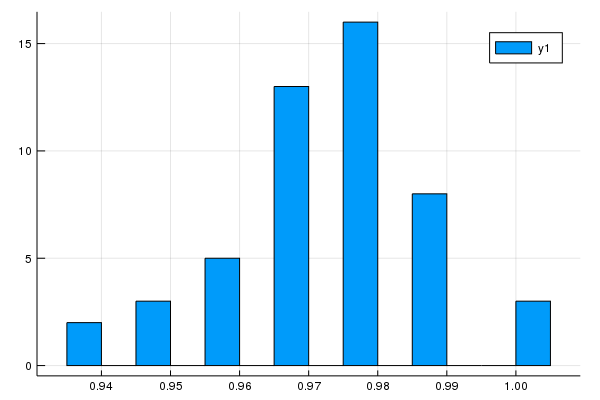In :
import Missings
using CSV
using Knet
using Plots
using Images, Colors
using Statistics
using StatsBase

WARNING: replacing module adv.

Out:
Main.adv

In :
include(Knet.dir("data","mnist.jl"))
xsize=784
ysize=10
train, test = mnistdata(xsize=(xsize,:))

Out:
(Data(Float32[0.0 0.0 … 0.0 0.0; 0.0 0.0 … 0.0 0.0; … ; 0.0 0.0 … 0.0 0.0; 0.0 0.0 … 0.0 0.0], UInt8[0x05 0x0a … 0x06 0x08], 100, 60000, false, 1:60000, false, (784, Colon()), (60000,), Array{Float32,N} where N, Array{UInt8,1}), Data(Float32[0.0 0.0 … 0.0 0.0; 0.0 0.0 … 0.0 0.0; … ; 0.0 0.0 … 0.0 0.0; 0.0 0.0 … 0.0 0.0], UInt8[0x07 0x02 … 0x05 0x06], 100, 10000, false, 1:10000, false, (784, Colon()), (10000,), Array{Float32,N} where N, Array{UInt8,1}))

## Initialize and train an MLP¶

In :
vals = (784, 64, 10)
accuracy(model, test)

adv.accuracy(model, test) = 0.1427
38.570436 seconds (10.93 M allocations: 28.193 GiB, 6.87% gc time)

Out:
0.978

In :
examples = []
for (x,y) in test
push!(examples, (x,y))
end


## Run an adversarial attack against the trained model on the test set¶

In :
# Choose a distance metric (recommended adv.L2_dist and adv.DSSIM)
# Initialize an attack algorithm with the desired parameters
# Attacks include:
# See function documentation in Adversarial.jl for more details on each function

example = examples
x0 = example[:, 1]
y0 = example
println("The sample is a $y0") target = 3 x1 = g(x0, model, target) pred = adv.predict_class(model, x1) dist = dist(x1, x0) println("The model classifies it as a$pred with a distance of \$dist from the original")

The sample is a 7
The model classifies it as a 3 with a distance of 7.840000000000003 from the original


## Display the original and adversarial images¶

In :
L = Int(√(length(x1)))
img1 = reshape(x0, (L,L))'
Gray.(img1)

Out:In :
img2 = reshape(x1, (L, L))'
Gray.(img2)

Out:## Run large scale tests and plot the success rates¶

In :
# This will run very slowly for adv.adv_LBFGS
success_rates = []
for i in 1:50